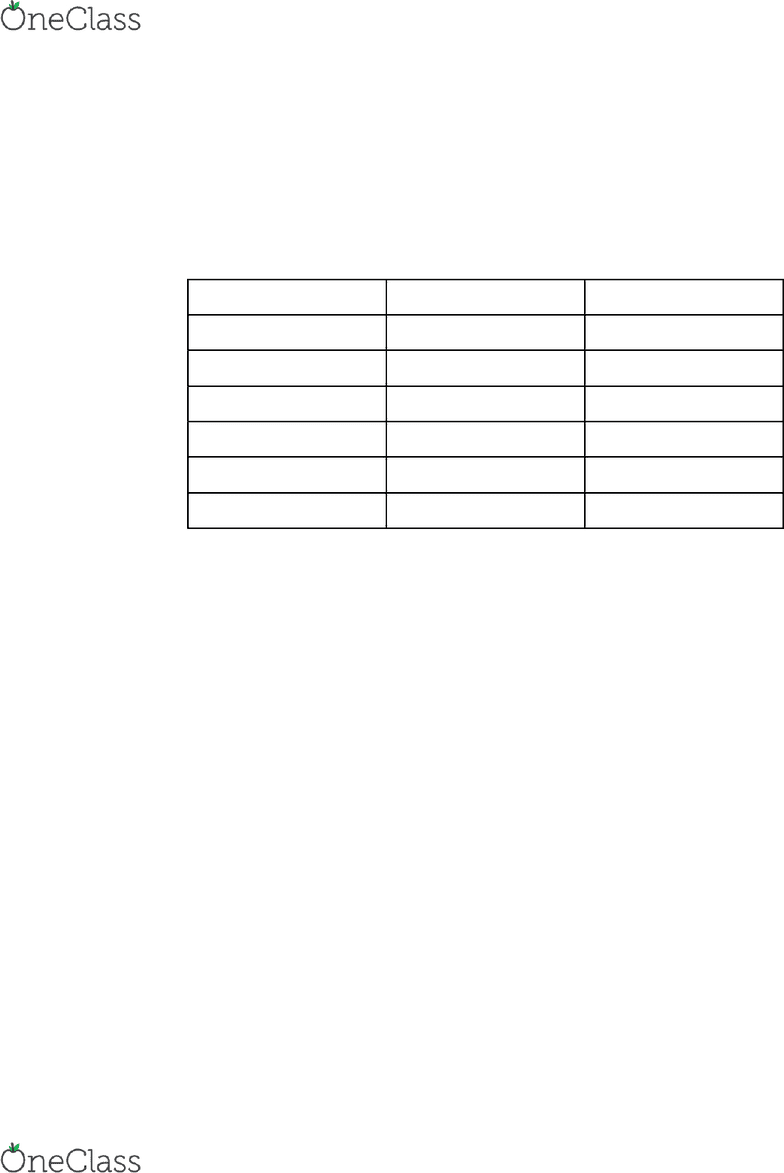Study Guides (400,000)
US (230,000)
Rutgers (3,000)
3:40 (100)
Guerra (3)
Study Guide

# 01:160:161 Study Guide - Fall 2018, Comprehensive Midterm Notes - Oxygen, Periodic Table, Molar Mass

This preview shows pages 1-3. to view the full 36 pages of the document.01:160:161
MIDTERM EXAM
STUDY GUIDE
Fall 2018

Only pages 1-3 are available for preview. Some parts have been intentionally blurred.Only pages 1-3 are available for preview. Some parts have been intentionally blurred.I. What is chemistry?
A. Chemistry - The study of matter
II. Measurements in science
A. A measured quantity must always include a unit
B. The english (or imperial) system has units such as the foot, gallon, pound, etc
C. The metric system includes units such as the meter, liter, kilogram, etc
D. Scientists have agreed to use the SI system (international system)
E.
Physical quantity
Name of unit
Symbol
Length
meter
m
Mass
kilogram
kg
Time
second
s
Temp
kelvin
k
Current
amper
amp
Amount
mole
mol
F. The SI base unit of mass is the kilogram (kg), but in chem the smaller gram (g) is
used
G. Atomic mass unit (u) is used to express the masses of atoms and other similar
sized objects
1. 1 u -    g
2. 1 g=  u (avogadro's number = mole)
H. MAss measures the amount of matter present in an object
I. Weight measures a force - the gravitational pull on an object (ie. what planet you
are on), in addition to the medium that surrounds it (buoyancy effect)
J. To temperature scales are commonly used in chem
1. The celsius scale (degrees C)
a) Freezing point of water at 1 atm - 0 degrees C
b) Boiling point of water at 1 atm - 100 degrees C
2. The kelvin scale (K)
a) The absolute scale
b) Lowest possible temp - 0 K (absolute zero)
K. The fahrenheit scale is common in the US but not often used in Chem
1. Freezing point of water at 1 atm - 32 degrees F
2. Boiling point of water at 1 atm is 212 degrees F
III. Examples
A. The temp of a sample is raised from 25 degrees C to 35 degrees C
1. What is the change in temp in degrees C? 10 degrees C
2. In K? 10 K
3. In fahrenheit? 18 degrees F
a) Degrees F = (9/5 * temp in degrees C) + 32 degrees F
find more resources at oneclass.com
find more resources at oneclass.com

Unlock to view full version

Only pages 1-3 are available for preview. Some parts have been intentionally blurred.B. SI derived units
1. Volume is derived by length
a) V=
2. SI unit for volume is a cubic meter (m^3)
3. More common SI for volume is a liter (L)
4. Example:
a) 1  =  =  = 1000 cm3
5. Density of a substance is the ratio of mass to volume (d=m/V)
6. Energy is the capacity to do work
7. Work ( W) is the change in energy that results when a force (F) is exerted
over a distance (d)
a) W = F * d
C. Energy conservation law
1. Total energy of an system is the sum of the three basic energy forms
a) E total = E k + V + E
2. Energy is measured in Joules (J)
a) 1 J = 1 kg*m2*s2
3. Energy is also measured in calories (cal) or kilowatt-hour (kWh)
a) 1 cal = 4.184 J
(1) 1 Cal = 1000 cal
b) 1 kWh = 3.6*106
4. Force is measured in Newtons (N)
a) F = m * a
b) 1 N = 1 kg*m2*s2
5. Pressure is measured in pascals (1 Pa = 1 N/m2) but other more common
units are atm, bar, psi, Torr, and mmHg
a) P = F/l2
(1) 1 atm = 101325 Pa = 1.01325 bar
(2) 1 atm = 14.7 psi
(3) 1 atm = 760 Torr = 760 mmHg
D. Scientific Notation
1. Scientific notation is a format that makes it easier to write and use very
big and very small numbers
2. Example:
a) 1.5 * 10-8 = .000000015
b) 26887 = 2.6887 * 10-4
c) .00000653 = 6.53 * 10-6
3. Multiplication and division with scientific notation
a) (3.5 * 10-8)(2.0 * 105) = 7.0 * 10-3